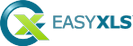The following listed chapters shows how to handle cells, cell values, formulas and how to use the calculation engine.
• Cell values
How to handle data in cells, strings, numeric values, dates, boolean values or error values for Excel worksheet
• Formulas
How to use formulas in sheets, names, data validation, charts and other Excel elements that use formulas
• Calculate formulas
How to calculate formulas in cells and use the calculation engine

#### EasyXLS Excel libraries:

##### .NET Excel Library
full .NET version to import, export or convert Excel files
##### COM+ Excel Component
full COM+ version to import, export or convert Excel files
##### Java Excel Library
full Java version to import, export or convert Excel files

#### File formats:

MS Excel 97 - 2003
MS Excel 2007 - 2019
MS Excel 2021
Office 365